Math & Beyond

# What is Series Completion? How to solve them?

879 views

 1 Introduction 2 What is Series Completion? 3 Finding the Missing Number(s) and wrong number(s) in the series 4 Finding the Missing Letter(s) in the alphabet series 5 Finding the Missing Figure(s) in the series 6 Learning Outcomes 7 Frequently Asked Questions (FAQs) 8 External References

06 January 2021

“We can’t solve the problems with the same thinking we used when we created them.”

-Albert Einstein

"Mathematics is a way to settle in the mind a habit of reasoning."

-John Locke

Mathematics is not a subject which only deals with the numbers. Instead, it is a path that helps human beings to develop logical and analytical skills. Logical reasoning or Logical thinking is a cognitive process of looking for reasons for beliefs, conclusions, actions, or feelings.

It is a process of concluding based on the evidence. Analytical skills are the ability to apply logical thinking to gather and analyze the information or data.

There are numerous ways to develop analytical skills. Some of them are

3. Working regularly on problem-solving skills
4. Studying the mechanism behind how things work.
5. Stimulating the brain by playing games.

## What is Series Completion? How to solve them?

In questions on number and alphabet Series Completion, a series of terms following a specific pattern will be given with some missing terms. The test-taker is expected to identify the pattern followed by the terms and extrapolate it to determine the missing term in the series.

What is Series Completion? How to solve them? is mentioned below in the Downloadable PDF.

## What is Series Completion?

A series of terms following a specific pattern will be given with some missing terms called as series completion. We need to identify the pattern followed by the terms and extrapolate it to determine the missing terms in the series.

It is a continuous sequence of numbers, figures, or words that are obtained by some particular previously defined rule. The next term of the series can be obtained by applying the predefined rule.

### Tricks to complete the series

1. Learn the squares of the natural number from 1 to 25.
2. Learn the cubes of the natural numbers from 1 to 20.
3. Check the sequence by analyzing the numbers, letters and by checking the difference, by dividing the numbers, multiplying the numbers with a constant number, etc.,
4. Concept of EJOTY i.e., E= 5th letter in English Alphabet, J=10th letter in the English Alphabet, O=15th letter in the English Alphabet, T=20th letter in the English Alphabet and Y=25th letter in the English Alphabet.
5. Concept of CFILORUX i.e., C = 3rd letter in English Alphabet, F = 6th letter in English Alphabet, I = 9th letter in English Alphabet, L = 12th letter in English Alphabet, O = 15th letter in English Alphabet, R =18th letter in English Alphabet, U = 21st letter in English Alphabet and X = 24th letter in English Alphabet.
6. Positioning of the letters from Right-Hand Side and Left-Hand Side.
7. Identifying the central letter/digit/symbol.
8. Identifying the patterns by separating it with a “/” or “space” after each iteration.

## Finding the Missing Number(s) and wrong number(s) in the series

Questions on the number series are common in almost all the competitive exams. These questions are based on the numerical concepts that follow a logical rule or predefined set of patterns which is based on the mathematical calculations. Therefore, to solve these kinds of questions, the analysis pattern is very important.

There are generally three kinds of questions that can be asked in number series. They are:

1. One of the numbers is wrongly placed in the series. We are asked to identify the wrong number.
2. One of the numbers is missing in the series. We are asked to find the missing number.
3. A complete number series is followed by an incomplete number series. We have to solve the incomplete series in the same pattern as the complete series is given.

Examples for Number Series

1. Choose the missing numbers to complete the series.

1, 4, 9, 16, 25, 36, ? ,?, 81, 100

(A) 36, 49
(B) 49, 64
(C) 25, 36
(D) 216, 125

Answer: Squares of the natural number. Therefore, the missing numbers are 72=49, 82=64.

 (B) 49, 64

2. Choose the missing numbers to complete the series.

3, 9, 27, 81, ?, ?

(A) 512, 343
(B) 729, 243
(C) 343, 512
(D) 243, 729

Answer: The sequence is 31 = 3, 32=9, 33=27, 34=81…Therefore the next numbers in the series are 35=243 and 36=729.

 (D) 243, 729

3.  A number is wrongly placed in the series. Identify the wrong number.

625, 576, 530, 484, 441, 400

(A) 441
(B) 530
(C) 576
(D) 400

Answer: Arranging the squares of the numbers in the descending order starting from 252=625, 242=576, etc. 232=529, but the option is given as 530 in the series.

 (B) 530

4. Complete the series. 10, 22, 46, 94, 190, ?

(A) 370
(B) 182
(C) 112
(D) 382

22*2+2=46

46*2+2=94

190*2+2=382

 (D) 382

5. Complete the series 3, 4, 8, 10, 13, 16, ?, ?

(A) 18, 22
(B) 14, 17
(C) 20, 27
(D) 19, 21

Answer: The given series has 2 different series in it.

Series 1: 3, 8, 13… in which the common difference is 5

Series 2: 4, 10, 16….in which the common difference is 6

The next numbers in the series are 18, 22

 (A) 18, 22

## Finding the Missing Letter(s) in the alphabet series

The easy way to solve these kinds of questions is to memorize the alphabets in English. There are 26 alphabets in English which can be divided into two parts.

The first half is from A-M which has 13 letters and the second half starts with N and ends with Z.

Examples for Alphabet Series

1. Complete the series: BAY, BEY, BIY, BOY, ?

(A) FOY
(C) GUY
(D) FUY

Answer: The middle letter is a vowel in the sequential arrangement A, E, I, O without changing the first and the last letters. Therefore, the answer is BUY.

2. Choose the missing series: AZ, CX, EV, GT, IR, ?

(A) KP
(B) KO
(C) KQ
(D) KS

Answer: The first letter in the series is the alternate alphabet from Left to Right. The second letter is the alternate alphabet from Right to left. Therefore, the next arrangement is KP.

 (A) KP

3. Complete the series: A, D, G, J, ?, ?

(A) M, Q
(B) N, P
(C) A, Z
(D) M, P

Answer: A+3=D; D+3=G; G+3=J; J+3=M; M+3=P

 (D) M, P

4. Choose the missing letter(s) to form the series:

ZY, WVU, TSRQ, ?, KJIHGF

(A) PONML
(B) ONML
(C) LKHM
(D) ABCD

Answer: The series has groups of alphabets in which the number of alphabet increases from one group to the next from left to right. 2 alphabets, 3 alphabets, 4 alphabets and the next option should have 5 letters.
Therefore, the answer is PONML, which is option (a).

 (A) PONML

5. Complete the series : _q_ppqq_pqqpp_qp

(A) pqpq
(B) qqpp
(C) pqqp
(d) qppq

Answer: Divide the series with a “/” pqqp/pqqp/pqqp/pqqp.

 (A) pqpq

## Finding the Missing Figure(s) in the series

The logic behind solving these questions is to have a clear observation. The arrangement is made on the basis of the predefined rule. So, we need to identify the rule and then solve the problem.

Example problems for Figure Series

1. Find the missing figure in the given series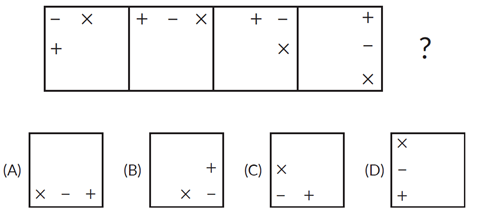Answer: Each side of the square is divided into three equal parts. The symbols are arranged in it and the symbols move towards right in every square by one step. The answer is Option (B).

 (B)

2. Find the missing figure in the series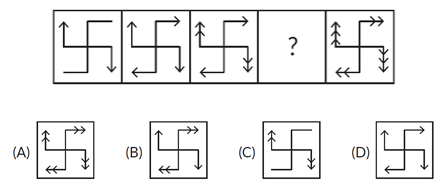(A)

3. Choose the missing figure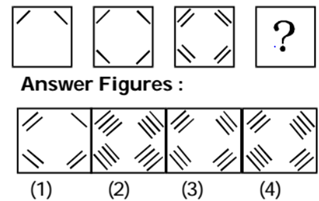Answer: The number of lines in the first box is 2, the number of lines in the second box is 4=22, the number of lines in the third box is 8=23. Therefore, the number of lines in the fourth box should be 24=16. So the answer is Option is (2).

 (2)

4. Choose the missing figure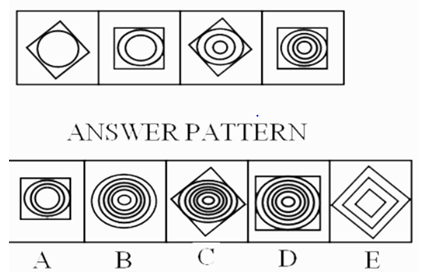Answer: The number of Circles inside the square keeps increasing from first pattern to the next. The square keeps rotating and in this sequence, the answer is Option C.

 (C)

5. Choose the missing figure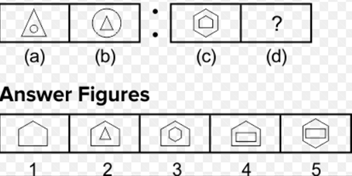Answer: Two groups with two images each. In the first group, the first image has the circle inside the triangle and the second image with triangle inside the circle. Similarly, the second group starts with pentagon inside hexagon and the second image would be hexagon inside pentagon. Therefore the answer is Option (3).

 (3)

## Learning Outcomes

1. Identify what series is and predict what should come next in the series.
2. Using various operations like addition, subtraction, multiplication and division to solve a problem.
3. Observing the figures carefully and matching the correct figure according to the problem.

Cuemath, a student-friendly mathematics and coding platform, conducts regular Online Live Classes for academics and skill-development, and their Mental Math App, on both iOS and Android, is a one-stop solution for kids to develop multiple skills. Understand the Cuemath fee structure and sign up for a free trial.

## What is figure series completion?

In these set of problems, a series of figures is given and the students are asked to select one of the figure from the set of options provided to continue the given sequence.

## What is number series?

In these set of problems, a series of numbers is given and the students are asked to select one of the correct number from the set of options provided to continue the given sequence.

-Written by Anusha Makam D R, Cuemath Teacher

## External References

Related Articles
GIVE YOUR CHILD THE CUEMATH EDGE
Access Personalised Math learning through interactive worksheets, gamified concepts and grade-wise courses